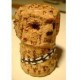1.Lasaro

In the last line, where “\leq0” should be replaced by “\geq 0”

•11 years later, this one line still hasn’t been corrected.

•Fixed now. Thanks 🙂

2.Cesar

Thanks guy! That’s really help

3.Yep, this helped me ! Thanks!

4.mathguy

Shouldn’t the integers be Z not I?

•You were right, thanks for the comment. I changed it.

Cheers,
Tom

5.Sean

Wow, awesome! Exactly what I needed: thanks!

6.This will really be helpful in writing my algebra and geometry blog, thanks.

Regards,

Mr. Pi

7.Enrique Argones Rúa

Why don’t you choose the more traditional notation \mathds{R}, \mathds{N}, etc.?

For using this you would have to include the package dsfont.

Cheers,

Enrique

8.Joe

THANK YOU! I’ve been digging around for an hour now looking for that. I can be picky about my fonts.

9.Joe

..I should have been more specific: thanks Enrique! The mathbb font is pretty well known, but the font for the more traditional number systems is hard to find.

10.•Hi,

Not sure if a number set symbol is commonly used for binary numbers. But try the following with any letter:

\usepackage{amssymb}
...
$\mathbb{B}$

Best, Tom.

11.one

Nice, thank you 🙂

12.Chewett

Thanks, very useful 🙂

13.Senthil

Thanks. Really its help to me.

14.Heni

Hi,how R^n write in Latex? THANK YOU ^^

•Hey Heni,

Thanks for your question. Is this what you were looking for?

\documentclass[11pt]{article}
\usepackage{amssymb}
\begin{document}
$\mathbb{R}^n$
\end{document}
•Joan

Though a minor difference, $\mathbb{R}^n$ produces a BOLD n as the dimension of R. Is there any way to make this n slimmer?

Thanks a lot.

•tom

Hi Joan,

How you perceive it might depend on the font used. In Computer Modern, the n doesn’t look bold in my opinion. Here’s what a bold n would look like, as compared to the normal font style in math mode:

\documentclass[11pt]{article}
\usepackage{amsfonts, amsmath, graphicx}
\begin{document}
$\mathbb{R}^{\boldsymbol n} \text{ vs. } \mathbb{R}^{n}$
\end{document}

Maybe you want to change the font size to make the letter n slimmer, and smaller obviously?

Also, I’d be curious to learn what configuration you used that made the letter thicker than what you would expect.

Cheers, Tom

15.Courtney

Hi! In the last line, the set of positive reals should be strictly R_>0, not R_≥0, which represents the nonnegative reals. The difference is subtle, but important 🙂

•Fixed! Thanks very much Courtney! Best, Tom.

16.m

Thanks! I found this very useful

•tom

17.I like 4285127

There also is W for whole, right?
(Btw, thx this helped a lot!)

•tom

Right, thanks for mentioning it! Tom

18.Thank you very much

19.Years later, your details provided just the solution I was looking for. Thanks.

•tom

Great to hear, thanks!

20.Petra

\documentclass{beamer}
\usepackage{amsfonts}
\begin{document}
\begin{frame}
\mathbb{Q}
\end{frame}
\end{document}

This isn’t working. Can you help me? It sais “\mathbb allowed only in math mode”

•tom

Hi Petra,

Thanks for the minimal working example. The command only works in math mode. So this should do the trick:

$\mathbb{Q}$

It wasn’t clear from the post and I’m going to change that. Thanks for bringing this up.

Best, Tom

21.NPandiya Rajan

Dear Friend, What is the symbol for composite numbers?

Send it to my mail_id

•tom

Hi there,

I’m not aware of a symbol for composite numbers.

Best, Tom

22.Thomas Pierre Nicolas Jean Brouard

hi it does not work for me for P. What can be wrong? I use the two packages

•tom

Hi Thomas,

Consider the example below. If this doesn’t help solving you problem, please provide a minimal working example to illustrate the issue.

\documentclass{article}
\usepackage{amssymb}
\begin{document}
$\mathbb{P}$
\end{document}

Best,
Tom

23.Andrew

How do I define my own sets so that they do not collide with the conventional ones? eg. P for a set of particles (not to mean a set of Primes) in Latex.

•tom

Hi Andrew,

I’m not sure I understand the question. Would you like to generate the same symbol with a different command or a similar, but different symbol?

24.Lisel

Hey Tom, thanks for your great work. I have an additional issue to solve. How can I represent this problem in a better way:

$\mathbb{R}^{m x n}$

as the output of the power term looks like: mxn when it would be great to have some space in between the symbols to show that its m x n and not a term mxn.

•tom

Hi Lisel,

Thanks for your question. I’d use the \times macro instead of “x”, as regular text gets printed in italics in math mode. The macro has some extra space left and right. If you need more, use can use the math spacing commands (\, or \: or \; — in increasing order).

HTH, Tom

\documentclass[11pt]{article}
\usepackage{amsfonts}
\begin{document}
$\mathbb{R}^{m\times{}n}$

$\mathbb{R}^{m\;\times\;n}$
\end{document}